Latest Banking jobs   »

# Quantitative Aptitude Quiz For IBPS RRB PO Prelims 2022- 14th June

Q1. P and Q together can complete a work in 24 days, while Q and R working together can complete the same job in 32 days. P and Q start the work and did it for 8 days, after that P left the work and R joined Q and after 12 more days, Q also left the work. Then, the remaining work is completed by R in 28 days. Find in how many days R will complete the work alone?
(a) 96 days
(b) 72 days
(c) 108 days
(d) 90 days
(e) 81 days

Q2. ‘A’ is 40% less efficient than ‘B’ who can do the same work in 20% less time than ‘C’. If A and B together can complete 80% of work in 12 days, then in how many days 60% of work can be completed by B and C together.
(a) 2 days
(b) 4 days
(c) 6 days
(d) 8 days
(e) 10 days

Q3. A Pipe can fill a tank in 2T hours. After one-fourth tank is filled, four more similar pipes are opened in the tank, find total time required to fill the tank completely?
(a) 0.8T
(b) 0.5T
(c) 0.6T
(d) 0.7T
(e) 0.9T

Q4. 8 men together can complete a work in 12 days. These 8 men started working together on the same work and have worked for 8 days, then X men more joined them which reduced the remaining work completion time by 33 ⅓%. Find the value of X?
(a) 3 men
(b) 4 men
(c) 5 men
(d) 6 men
(e) 2 men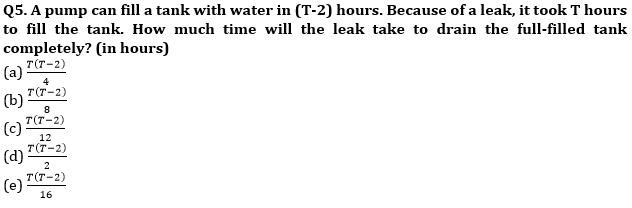Q6. Abhi, Roly and Bitu can complete a work in 60 days working together. If Abhi and Bitu together are twice efficient than Roly and Abhi and Roly together are thrice efficient than Bitu then in how many days Abhi alone complete the work?
(a) 120 days
(b) 96 days
(c) 84 days
(d) 144 days
(e) 110 days

Q7. A and B together can complete 4/7 th of a work in 16 days. If efficiency of B is 75% of that of A, then in how many days A alone will complete the whole work.
(a) 42 days
(b) 36 days
(c) 52 days
(d) 49 days
(e) 55 days

Q8. Two Containers A and B of equal capacities contains mixture of milk and water, in container A water is 40% more than the milk and in container B milk in 300% of the water. If both containers poured into an empty Container C then the quantity of milk in container C becomes 80 liters more than the quantity of the water in it. find the quantity of water in container B?
(a) 60 L
(b) 50 L
(c) 40 L
(d) 80 L
(e) 90 L

Q9. A and B together can complete a work in 12 days and A alone can complete the same work in 30 days. If they start the same work by working on alternate days starting with A, then find the required time to Complete the work?
(a) 20 days
(b) 30 days
(c) 24 days
(d) 25 days
(e) 22 days

Q10. A water tank normally takes 9 hours to be filled by a tap but because of the leak, it takes another 3 hours. In how many hours will the leak empty a full water tank?
(a) 36 hours
(b) 72 hours
(c) 30 hours
(d) 32 hours
(e) 28 hours

Q11. Tap X can fill a tank in 12 minutes and tap Y can fill the same tank is 15 minutes, another tap Z can empty the tank in 10 minutes. If tap X and tap Y are opened & after 6 minutes tap Z is also opened. Then find the total time taken to fill the tank completely?
(a) 8 minutes
(b) 4 minutes
(c) 2 minutes
(d)12 minutes
(e)6 minutes

Q12. A & B can do a piece of work together in 24 days and C’s efficiency is half of A’s efficiency. If all three work together, they can complete the same work in 20 days, then find how many days are required to complete the work, if B and C work together?
(a) 45 days
(b) 42 days
(c) 30 days
(d) 48 days
(e) 44 days

Q13. Abhi takes 40% more time than Ashu to complete a work. If Ashu works for X days & after that the remaining work is completed by Abhi in (x + 4) days than ratio of work done by Ashu and by Abhi is 7 : 9. Find in how many days Abhi will complete the work alone?
(a) 26 days
(b) 14 days
(c) 24 days
(d) 12 days
(e) 16 days

Q14. Time taken by Rahul and Riya together to complete a work is 2 days less than the time taken by Riya alone to complete the same work. If ratio of efficiency of Rahul and Riya is 1:2 respectively, then find in how many days Riya alone can complete the same work?
(a) 8 days
(b) 7 days
(c) 6 days
(d) 5 days
(e) 4 days

Q15. P, Q and R can complete a work in 12, 15 and 25 days respectively. They received Rs. 13,500 for the completion of work. P and Q started the work together but after 2 days, Q left the work and P left 7 days before the completion of the work. The remaining work is completed by R working for the last 5 days. Then, find the share of P.
(a) Rs. 8100
(b) Rs. 9500
(c) Rs. 8550
(d) Rs. 9000
(e) Rs. 8800

Solutions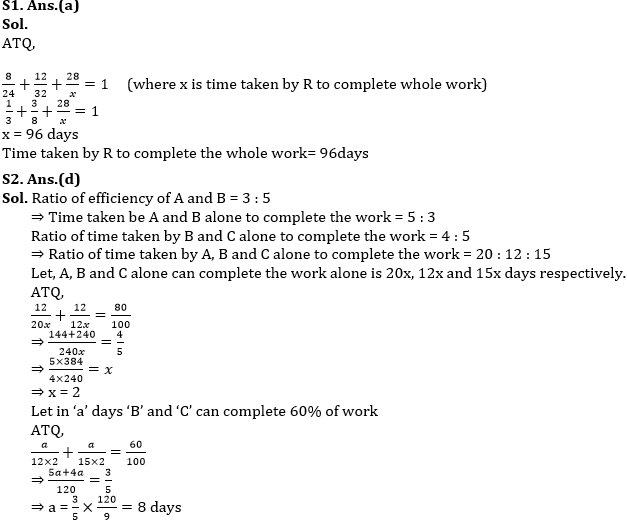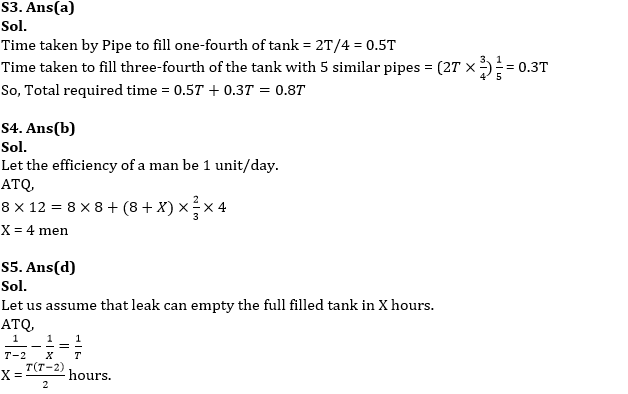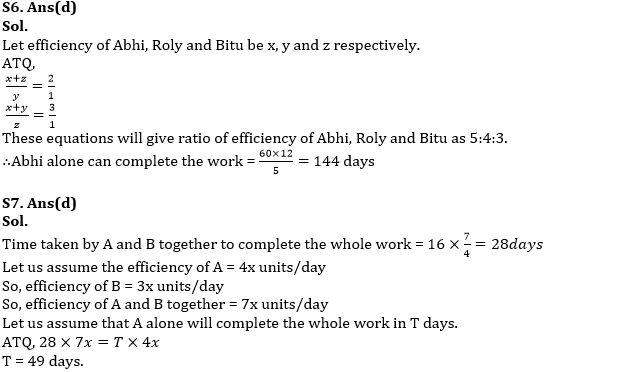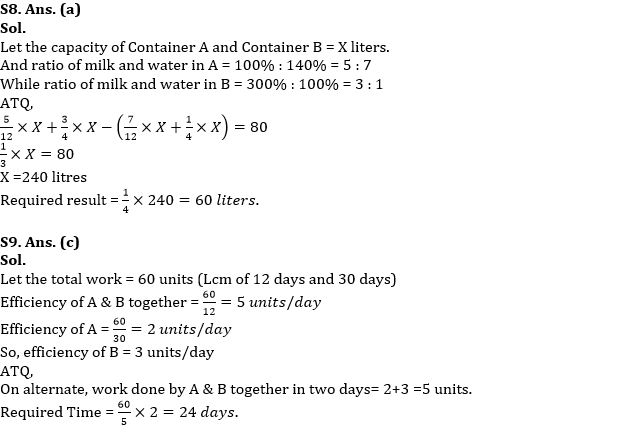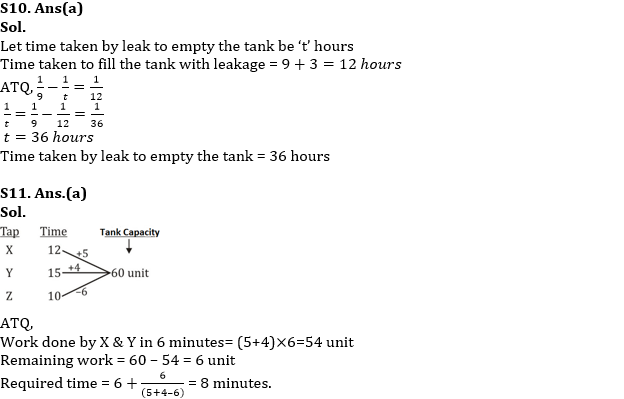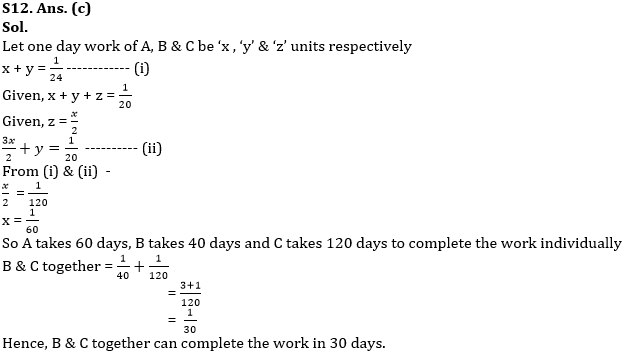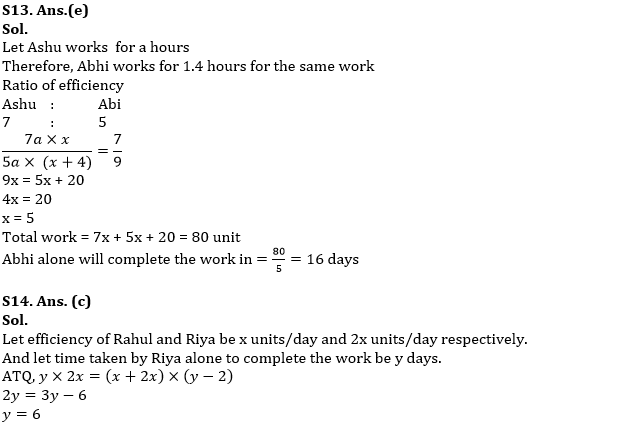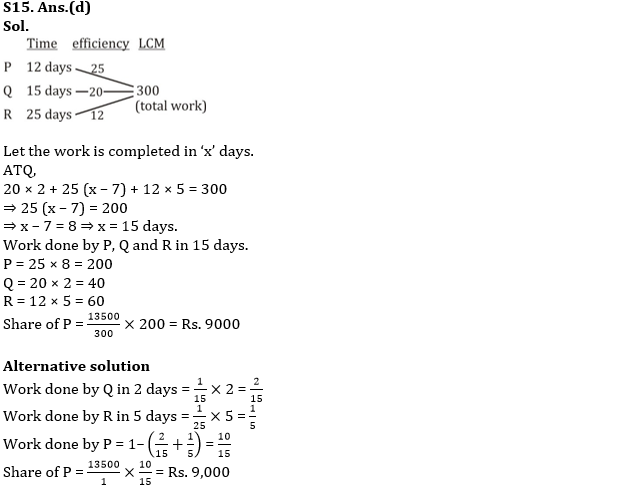#### Congratulations!